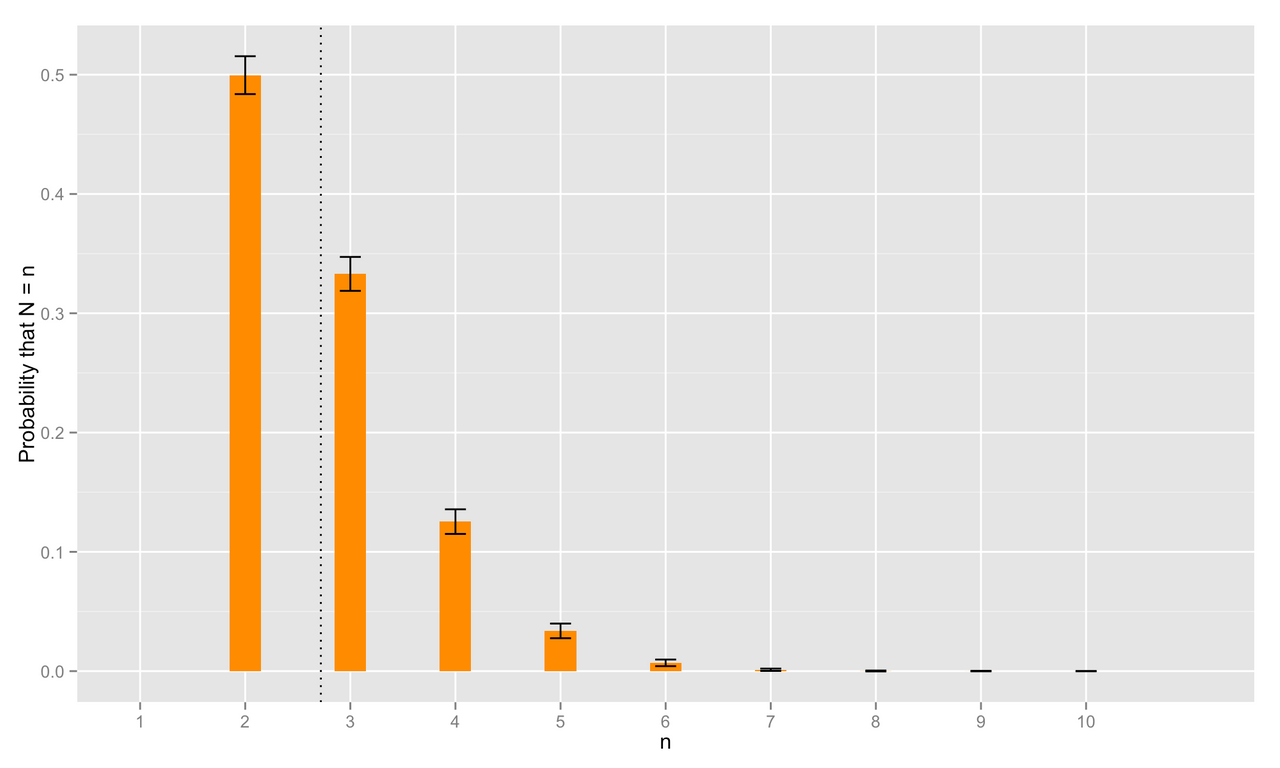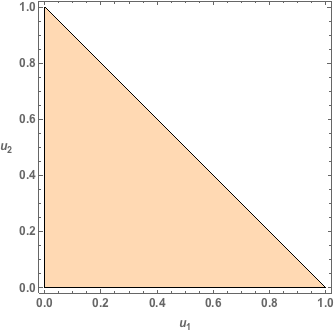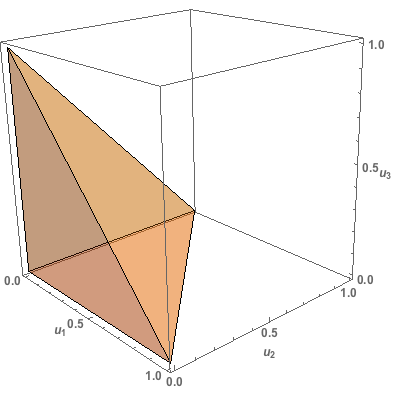## Neal Grantham

[email protected]

# The Sum Over 1 Problem

I recently came across this fun probability problem which I’ve dubbed the ‘Sum Over 1’ problem.

It goes like this:

On average, how many random draws from the interval [0, 1] are necessary to ensure that their sum exceeds 1?

To answer this question we’ll use some probability theory (probability rules, distribution functions, expected value) and a dash of calculus (integration).

## Let’s Begin!

Let $$U_i$$ follow a Uniform distribution over the interval $$[0, 1]$$, where $$i = 1, 2, \ldots$$ denotes the $$i^{\text{th}}$$ draw.

Let $$N$$ denote the number of draws such that $$\sum_{i=1}^{N} U_i > 1$$ and $$\sum_{i=1}^{N-1} U_i \leq 1$$. Thus, $$N$$ is a discrete random variable taking values $$n=2,3,\ldots$$ ($$n=1$$ is impossible because no single draw from $$[0, 1]$$ can exceed 1).

We seek the long-run average, or expected value, of $$N$$ denoted $$E(N)$$. A good first step for a numerical problem like this is to utilize computer simulations to point us in the right direction.

## Simulations with R

Let’s generate, say, 20 random draws from $$[0,1]$$, $$(u_1, \ldots, u_{20})$$.

draws <- runif(20)  # vector of u_1, ..., u_20


Consider the partial sums of these draws $$(u_1, u_1 + u_2, \sum_{i=1}^3 u_i, \ldots, \sum_{i=1}^{20} u_i)$$.

If we index this partial sum vector from 1 up to 20, then $$n$$ here is simply the index of the first component of this vector whose value exceeds 1.

The find_n function accomplishes this for any vector of random draws u:

find_n <- function(u) {
which(cumsum(u) > 1)  # which partial sum first exceeds 1?
}

n <- find_n(draws)
draws[1:n]

##  0.2858056 0.1689087 0.6259122


In this instance, it took 3 random draws from $$[0, 1]$$ to sum over 1. We can sample from the probability distribution of $$N$$ by repeating this process many more times. The sample_from_N function takes m samples from the distribution of $$N$$:

sample_from_N <- function(m) {
# m-by-20 matrix of random [0,1] draws
draws <- matrix(runif(m * 20), ncol = 20)
# for each row of 20 draws, find n
n.many <- apply(draws, 1, find_n)
# estimate P(N = n), n = 2, 3, ..., 10
# (truncated b/c probs are miniscule for n > 10)
estimated.probs <- table(factor(n.many, levels = 2:10)) / m
return(estimated.probs)
}

m <- 1000
approx.N <- sample_from_N(m)  # approximate prob. dist'n of N
approx.N

##
##     2     3     4     5     6     7     8     9    10
## 0.514 0.330 0.121 0.026 0.005 0.004 0.000 0.000 0.000


So, we estimate $$P(N = 2)$$ by $$\hat{P}(N = 2) = 0.514$$, $$P(N = 3)$$ by $$\hat{P}(N = 3) = 0.33$$, and so forth.

These are just point estimates for $$P(N = n)$$, but we can estimate their standard errors as well by running sample_from_N many more times. The approximate probability distribution of $$N$$ is depicted below.The dotted line here marks the estimated expected value of $$N$$, $$\hat{E}(N) = \sum_{n=2}^{\infty} n\hat{P}(N=n) \approx 2.71962$$. This value is tantalizingly close to the mathematical constant $$e\approx 2.7183$$ known as Euler’s number1.

Could the answer to our probability puzzle truly be $$e$$?

## Proof

Let’s start small by finding the probability that $$N=2$$. The probability that it takes exactly 2 random draws from $$[0, 1]$$ for their sum to exceed 1 is simply the complement of the probability that the sum of our 2 draws fall short of exceeding 1. That is,

\begin{equation} P(N = 2) = P(U_1 + U_2 > 1) = 1 - P(U_1 + U_2 \leq 1). \end{equation}

We can interpret the probability that $$U_1 + U_2 \leq 1$$ geometrically. Since $$U_1$$ and $$U_2$$ are Uniform random variables on $$[0, 1]$$, the point $$(U_1, U_2)$$ can arise anywhere in the unit square with equal probability. Thus, $$P(U_1 + U_2 \leq 1)$$ corresponds exactly to the proportion of the unit square shaded in the following image.This shaded region is referred to as a 2-dimensional unit simplex2 with area

$\begin{equation} P(U_1 + U_2 \leq 1) = \int_0^{1}\int_0^{1-u_2}du_1du_2 = \int_0^{1}(1-u_2)du_2 = 1/2 \end{equation}$

So $$P(N=2) = 1 - 1/2 = 1/2$$, which agrees nicely with our simulation estimate $$\hat{P}(N = 2) = 0.514$$.

Now for the probability that $$N=3$$, it is tempting to proceed by finding the exact probability

$\begin{equation} P(N = 3) = P(U_1 + U_2 + U_3 > 1, U_1 + U_2 \leq 1), \end{equation}$

but dealing with both inequality constraints simultaneously is annoying. It is helpful to recognize that $$P(N = 3) = P(N \leq 3) - P(N = 2)$$ where

$\begin{equation} P(N \leq 3) = P(U_1 + U_2 + U_3 > 1) = 1 - P(U_1 + U_2 + U_3 \leq 1). \end{equation}$

Similar to the previous case, the probability that the sum of three random draws from [0,1], $$U_1, U_2,$$ and $$U_3$$, does not exceed 1 is given by the proportion of the unit cube shaded in the following image.This shaded region is a 3-dimensional unit simplex with volume

\begin{align} P(U_1 + U_2 + U_3 \leq 1) &= \int_0^{1}\int_0^{1-u_3}\int_0^{1-u_3-u_2}du_1du_2du_3 \\ &= \int_0^{1}\frac{1}{2}\left[1 - 2u_3 + u_3^{2}\right]du_3 \\ &= 1/6. \end{align}

So $$P(N=3) = P(N\leq 3) - P(N=2) = (1 - 1/6) - 1/2 = 1/3$$ which again agrees with our simulation estimate of $$\hat{P}(N = 3) = 0.33$$.

Foregoing a formal proof, we can generalize our results on the unit simplex: the $$n$$-dimensional unit simplex has “volume” given by $$1 / n!$$. For our purposes, $$P(N \leq n) = 1 - 1/n!$$. Thus, for any $$n\geq 2$$,

\begin{align} P(N = n) &= P(N \leq n) - P(N \leq n - 1) \\ &= \left[1 - \frac{1}{n!}\right] - \left[1 - \frac{1}{(n-1)!}\right] \\ &= \frac{n - 1}{n!}. \end{align}

We’ve got everything we need to find the expected value of $$N$$.

\begin{align} E(N) = \sum_{n = 2}^\infty n P(N = n) = \sum_{n = 2}^\infty n\left(\frac{n - 1}{n!}\right) = \sum_{n = 2}^\infty \frac{1}{(n - 2)!} = \sum_{n = 0}^\infty \frac{1}{n!} \end{align}

Recall the exponential series is given by $$e^{x} = \sum_{n=0}^{\infty} \frac{x^{n}}{n!}$$.

Thus, $$E(N)$$ is equivalently $$e$$.

Yes that’s right: we expect, on average, $$e$$ random draws from the interval $$[0, 1]$$ to ensure their sum exceeds 1!

1. A convenient way to remember $$e$$: Take 0.3 from 3 (2.7) followed by the election year of former U.S. president (and horrible human being) Andrew Jackson twice in a row (1828). That is, $$e \approx 2.718281828$$. Okay, maybe it’s not that helpful, but you’re slightly more prepared for American presidential history trivia!

2. An $$n$$-dimensional unit simplex is the region of $$n$$-dimensional space for which $$x_1 + x_2 + \cdots + x_n \leq 1$$ and $$x_i > 0, i = 1, 2, \ldots, n$$. A unit simplex in one dimension (1D) is boring; it’s just the unit interval $$[0, 1]$$. In 2D, the unit simplex takes the form of a triangle bounded by $$x_1 + x_2 \leq 1, x_1 > 0, x_2 > 0$$. In 3D, we get a tetrahedron, in 4D a hypertetrahedron, and in $$n$$ dimensions — well, an $$n$$-dimensional simplex.

October 30, 2014  @nsgrantham